In this page, we are providing Online Education for Magnetic Effects of Electric Current Class 10 Extra Questions and Answers Science Chapter 13 pdf download. NCERT Extra Questions for Class 10 Science Chapter 13 Magnetic Effects of Electric Current with Answers will help to score more marks in your CBSE Board Exams. https://ncertmcq.com/extra-questions-for-class-10-science/

## Online Education for Class 10 Science Chapter 13 Extra Questions and Answers Magnetic Effects of Electric Current

Extra Questions for Class 10 Science Chapter 13 Magnetic Effects of Electric Current with Answers Solutions

### Extra Questions for Class 10 Science Chapter 13 Very Short Answer Type

Magnetic Effect Of Electric Current Class 10 Extra Questions And Answers Question 1.
Define magnetic effect of electric current.
The production of magnetic field around a conductor when electric current is passed through it.

Magnetic Effect Of Electric Current Extra Questions Question 2.
What is fuse?
Fuse is the most important safety device, used for protecting the circuits due to short-circuiting or overloading of the circuits.

Extra Questions Of Magnetic Effect Of Electric Current Question 3.
What is the pattern of magnetic field around a current carrying conductor?
The magnetic field around a current carrying conductors forms a pattern of concentric circles.

Magnetic Effects Of Electric Current Class 10 Extra Questions Question 4.
Draw an appropriate schematic diagram showing common domestic circuits and discuss the importance of fuse.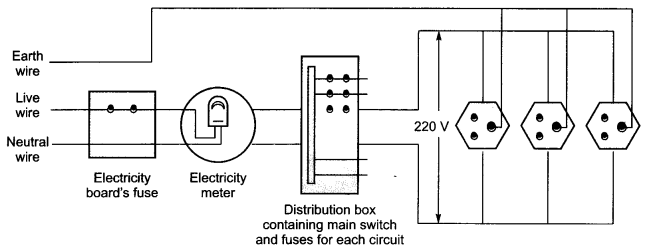### Extra Questions for Class 10 Science Chapter 13 Short Answer Type

Class 10 Science Chapter 13 Extra Questions Question 1.
What is electric motor? Write down its principle of working.
Electric motor: An electric motor is a rotating device that converts electrical energy to mechanical energy.
Principle: A current-carrying conductor when placed in a magnetic field experiences a force.

Magnetic Effects Of Electric Current Extra Questions Question 2.
What is galvanometer?
A galvanometer is an instrument that can detect the presence of a current in a circuit.
The pointer remains at zero (the centre of the scale) for zero current flowing through it.
It can deflect either to the left or to the right of the zero mark depending on the direction of current.Class 10 Magnetic Effect Of Electric Current Extra Questions Question 3.
Describe domestic electric circuits.
Domestic Electric circuits: In our houses, we receive AC electric power of 220 V with a frequency of 50 Hz. One of the wires in this supply is with red insulation, called live wire. The other one is of black insulation, which is a neutral wire. The potential difference between the two is 220 V.

The third is the earth wire that has green insulation and this is connected to a metallic body deep inside the earth. It is used as a safety measure to ensure that any leakage of current to a metallic body of an appliance does not give any severe shock to the user.

Ch 13 Science Class 10 Extra Questions Question 4.
What will happen if a current carrying conductor is placed in magnetic field? How can the direction of magnetic field be found out?
A current-carrying conductor when placed in a magnetic field experiences a force.
If the direction of the field and that of the current are mutually perpendicular to each other, then the force acting on the conductor will be perpendicular to both as given by Fleming’s left-hand rule.

Magnetic Effect Of Electric Current Class 10 Extra Questions Question 5.
What does the direction of thumb indicate in the right-hand thumb rule? In what ways this rule is different from Fleming’s left-hand rule?
The thumb indicates the direction of current in the straight conductor held by curled fingers, whereas Fleming’s left-hand rule gives the direction of force experienced by current carrying conductor placed in an external magnetic field.

Extra Questions Of Chapter 13 Class 10 Science Question 6.
What is the difference between a direct current and an alternating current? How many times does AC used in India change direction in one second?
Direct current always flows in one direction but the alternating current reverses its direction periodically. The frequency of AC in India is 50 Hz and in each cycle it alters direction twice. Therefore, AC changes direction 2 × 50 = 100 times in one second.

Class 10 Magnetic Effects Of Electric Current Extra Questions Question 7.
What is the role of fuse, used in series with any electrical appliance? Why should a fuse with defined rating not be replaced by one with larger rating?
Fuse is used for protecting appliances due to short-circuiting or overloading. The fuse is rated for a certain maximum current and blows off when a current more than the rated value flows through it. If a fuse is replaced by one with larger ratings, the appliances may get damaged while the protecting fuse does not burn off. This practice of using fuse of improper rating should always be avoided.

Chapter 13 Science Class 10 Extra Questions Question 8.
On which factors does the magnetic field produced by a current carrying conductor at a given point depend?
The magnetic field produced by a given current decreases as the distance from it increases.
The magnitude of the magnetic field produced at a given point increases as the current through the wire increases.

Extra Questions Of Magnetic Effect Of Electric Current Class 10 Question 9.
What is electric generator? Write down its principle of working.
Electric Generator: A generator converts mechanical energy into electrical energy.
Principle: It works on the basis of electromagnetic induction. AC generator produces AC current and DC generator produces DC current.

Extra Questions On Magnetic Effect Of Electric Current Question 10.
What is difference between AC and DC? Write down advantage of AC over DC?
The difference between the direct and alternating currents is that the direct current always flows in one direction, whereas the alternating current reverses its direction periodically.

In India, the AC changes direction after every 1/100 second, that is, the frequency of AC is 50 Hz. An important advantage of AC over DC is that electric power can be transmitted over long distances without much loss of energy.

Class 10 Chapter 13 Science Extra Questions Question 11.
With the help of a labelled circuit diagram illustrate the pattern of field lines of the magnetic field around a current carrying straight long conducting wire. How is the right hand thumb rule useful to find direction of magnetic field associated with a current carrying conductor?
Right hand thumb rule states that if a current carrying straight conductor is held in the right hand with the thumb pointing towards the direction of current, then the fingers will wrap around the conductor in the direction of the field lines of the magnetic field.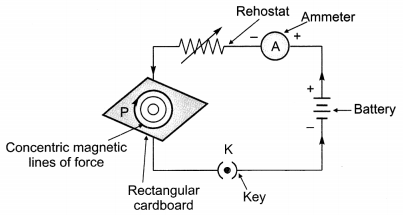Extra Question Of Magnetic Effect Of Electric Current Question 12.
Describe the activity that shows that a current-carrying conductor experiences a force perpendicular to its length and the external magnetic field. How does Fleming’s left-hand rule help us to find the direction of the force acting on the current carrying conductor?

• Take a small aluminium rod AB (of about 5 cm). Using two connecting wires, suspend it horizontally from a stand.
• Place a strong horse-shoe magnet in such a way that the rod lies between the two poles with the magnetic field directed upwards. For this put the north pole of the magnet vertically below and south pole vertically above the aluminium rod.
• Connect the aluminium rod in series with a battery, a key and a rheostat.
• Now pass a current through the aluminium rod from end B to end A.
• It is observed that the rod is displaced towards the left. You will notice that the rod gets displaced.
• Reverse the direction of current flowing through the rod and observe the direction of its displacement. It is now towards the right.

The displacement of the rod in the above activity suggests that a force is exerted on the current-carrying aluminium rod when it is placed in a magnetic field. According to Fleming’s left hand rule stretch the thumb, forefinger and central finger of your left hand such that they are mutually perpendicular.

If the forefinger points in the direction of magnetic field and the central in the direction of current, then the thumb will point in the direction of motion or force acting on the conductor.### Extra Questions for Class 10 Science Chapter 13 Long Answer Type

Class 10 Science Ch 13 Extra Questions Question 1.
Write down a short note on magnetic field produced by a solenoid.
Magnetic field due to a solenoid
Solenoid: A coil of many circular turns of insulated copper wire wrapped closely in the shape of a cylinder is called a solenoid.

• The pattern of the magnetic field around a current carrying solenoid is same as that of a bar magnet. One end of the solenoid behaves as a magnetic North pole, while the other behaves as a South pole.
• The field lines inside the solenoid are in the form of parallel straight lines.
• The field is uniform inside the solenoid.
• A strong magnetic field produced inside a solenoid can be used to magnetise a piece of magnetic material, like soft iron, when placed inside the coil. The magnet so formed is called an electromagnet.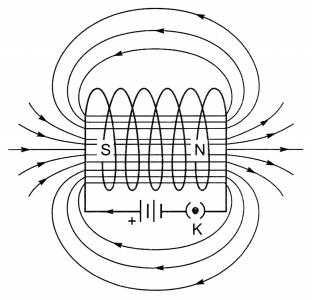Field lines of the magnetic field through and around a current carrying solenoidA current-carrying solenoid coil is used to magnetise steel rod inside it-an electromagnet

Extra Questions On Magnetic Effects Of Electric Current Class 10 Question 2.
Describe the magnetic field produced due to current-carrying circular loop.
Magnetic field due to current-carrying circular loop

• At the centre of the current carrying loop the magnetic field appears to be a straight line.
• The magnetic field produced by a current carrying wire at a given point depends directly on current passing through it.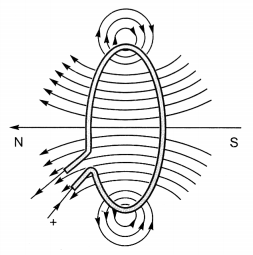Magnetic field lines of the field produced by a current-carrying circular loop

Question 3.
Define electromagnetic induction. Explain the ways by which magnetic field linked through a coil can be changed.
Electromagnetic Induction: The phenomenon of electromagnetic induction is the production of induced current in a coil placed in a region where the magnetic field changes with time.

1. The magnetic field may change due to relative motion between the coil and the magnet.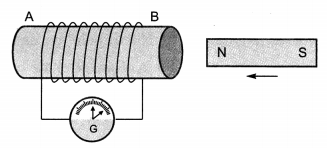Moving a magnet towards a coil sets up a current in the coil circuit, as indicated by deflection in the galvanometer needle

2. If the coil is placed near a current carrying conductor, the magnetic field may change either due to change in the current through the conductor or due to the relative motion between the coil and the conductor.current is included in coil – 2 when current in coil – 1 changed

Question 4.
Define magnetic field and magnetic field lines. Write down the properties of magnetic field lines.
Magnetic field:
The region surrounding a magnet, in which the force of the magnet can be detected, is said to have a magnetic field.

Magnetic field lines:
Magnetic field lines are the imaginary lines drawn in a magnetic field along which a north magnetic pole would move.

Properties of magnetic field line:

• Magnetic field lines are closed curves.
• The relative strength of the magnetic field is shown by degree of closeness of the field lines.
• No two field lines can cross each other as at the point of intersection the compass needle would point towards two directions, which is not possible.### Magnetic Effects of Electric Current HOTS Questions With Answers

Question 1.
AB is a current carrying conductor in the plane of the paper as shown in the Figure. What are the directions of magnetic fields produced by it at points P and Q? Given r1 > r2, where will the strength of the magnetic field be larger?
Into the plane of paper at P and out of it at Q. The strength of the magnetic field is larger at the point located closer i.e., at Q.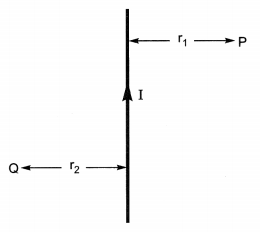Question 2.
Under what conditions permanent electromagnet is obtained if a current carrying solenoid is used? Support your answer with the help of a labelled circuit diagram.  [NCERT Exemplar]

1. The current through the solenoid should be direct current.
2. The rod inside is made of a magnetic material such as steel.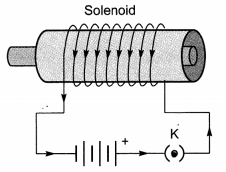Question 3.
A magnetic compass shows a deflection when placed near a current carrying wire. How will the deflection of the compass get affected if the current in the wire is increased? Support your answer with a reason.
The deflection increases. The strength of magnetic field is directly proportional to the magnitude of current passing through the straight conductor.

Question 4.
It is established that an electric current through a metallic conductor produces a magnetic field around it. Is there a similar magnetic field produced around a thin beam of moving (i) alpha particles, (ii) neutrons? Justify your answer.
(i) Yes, Alpha particles being positively charged constitute a current in the direction of motion.
(ii) No, the neutrons being electrically neutral does not constitute any current.

Question 5.
Why does a magnetic compass needle pointing North and South in the absence of a nearby magnet get deflected when a bar magnet or a current carrying loop is brought near it. Describe some salient features of magnetic lines of field concept.